# In the laboratory, a general chemistry student measured the pH of a 0.579 M aqueous solution...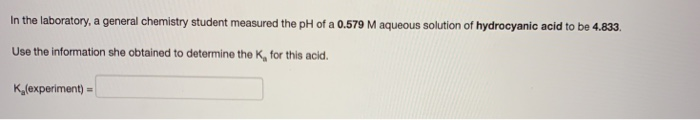In the laboratory, a general chemistry student measured the pH of a 0.579 M aqueous solution of hydrocyanic acid to be 4.833. Use the information she obtained to determine the K, for this acid. K(experiment)

use:

pH = -log [H+]

4.833 = -log [H+]

[H+] = 1.469*10^-5 M

HA dissociates as:

HA -----> H+ + A-

0.579 0 0

0.579-x x x

Ka = [H+][A-]/[HA]

Ka = x*x/(c-x)

Ka = 1.469*10^-5*1.469*10^-5/(0.579-1.469*10^-5)

Ka = 3.727*10^-10

#### Earn Coin

Coins can be redeemed for fabulous gifts.

Similar Homework Help Questions
• ### In the laboratory, a general chemistry student measured the pH of a 0.506 M aqueous solution...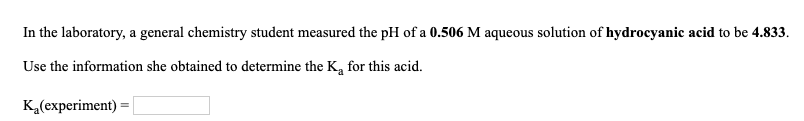In the laboratory, a general chemistry student measured the pH of a 0.506 M aqueous solution of hydrocyanic acid to be 4.833. Use the information she obtained to determine the K, for this acid. K (experiment) In the laboratory, a general chemistry student measured the pH of a 0.540 M aqueous solution of benzoic acid, CH3COOH to be 2.218. Use the information she obtained to determine the K, for this acid. K (experiment)

• ### 15.) a.)In the laboratory, a general chemistry student measured the pH of a 0.376 M aqueous...

15.) a.)In the laboratory, a general chemistry student measured the pH of a 0.376 M aqueous solution of nitrous acid to be 1.872. Use the information she obtained to determine the Ka for this acid. Ka(experiment) = b.)In the laboratory, a general chemistry student measured the pH of a 0.376 M aqueous solution of nitrous acid to be 1.870. Use the information she obtained to determine the Ka for this acid. Ka(experiment) = c.)In the laboratory, a general chemistry student...

• ### In the laboratory, a general chemistry student measured the pH of a 0.488 M aqueous solution...

In the laboratory, a general chemistry student measured the pH of a 0.488 M aqueous solution of hydrofluoric acid to be 1.711. Use the information she obtained to determine the Ka for this acid. Ka(experiment) = In the laboratory, a general chemistry student measured the pH of a 0.488 M aqueous solution of benzoic acid, C6H5COOH to be 2.273. Use the information she obtained to determine the Ka for this acid. Ka(experiment) = In the laboratory, a general chemistry student...

• ### In the laboratory, a general chemistry student measured the pH of a 0.430 M aqueous solution...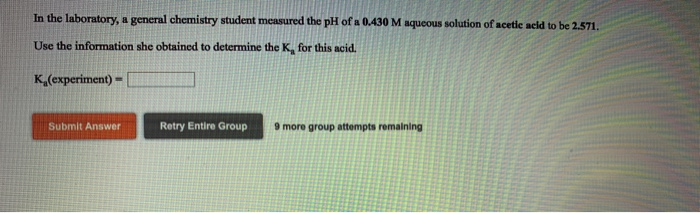In the laboratory, a general chemistry student measured the pH of a 0.430 M aqueous solution of acetie acid to be 2.571. Use the information she obtained to determine the K, for this acid. Ka(experiment)- Submit Answer Retry Entire Group 9 more group attempts remaining In the laboratory a student measures the percent ionization of a 0.430 M solution of acetic acid to be 0626 %. Calculate value of Ka from this experimental data. Ka-l In the laboratory, a general...

• ### In the laboratory, a general chemistry student measured the pH of a 0.508 M aqueous solution...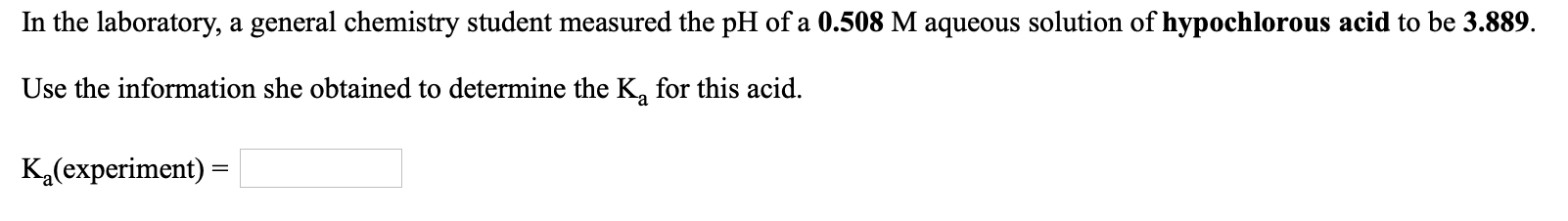In the laboratory, a general chemistry student measured the pH of a 0.508 M aqueous solution of hypochlorous acid to be 3.889. Use the information she obtained to determine the K, for this acid. K (experiment) =

• ### 1)In the laboratory, a general chemistry student measured the pH of a 0.360 M aqueous solution...

1)In the laboratory, a general chemistry student measured the pH of a 0.360 M aqueous solution of triethylamine, (C2H5)3N to be 12.120.   Use the information she obtained to determine the Kb for this base.   Kb(experiment) =​ 2)In the laboratory, a general chemistry student measured the pH of a 0.360 M aqueous solution of aniline, C6H5NH2 to be 9.198.   Use the information she obtained to determine the Kb for this base.   Kb(experiment) =​

• ### In the laboratory, a general chemistry student measured the pH of a 0.352 M aqueous solution of acetylsalicylic acid (a...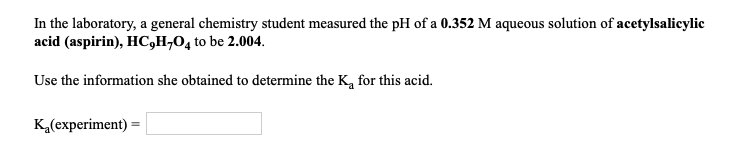In the laboratory, a general chemistry student measured the pH of a 0.352 M aqueous solution of acetylsalicylic acid (aspirin), HC,H704 to be 2.004. Use the information she obtained to determine the K, for this acid. K(experiment) =

• ### In the laboratory, a general chemistry student measured the pH of a 0.392 M aqueous solution...

In the laboratory, a general chemistry student measured the pH of a 0.392 M aqueous solution of formic acid, HCOOH to be 2.059.   Use the information she obtained to determine the Ka for this acid.   Ka(experiment) =

• ### In the laboratory, a general chemistry student measured the pH of a 0.464 M aqueous solution...

In the laboratory, a general chemistry student measured the pH of a 0.464 M aqueous solution of formic acid, HCOOH to be 2.057.   Use the information she obtained to determine the Ka for this acid.   Ka(experiment) =

• ### In the laboratory, a general chemistry student measured the pH of a 0.370 M aqueous solution...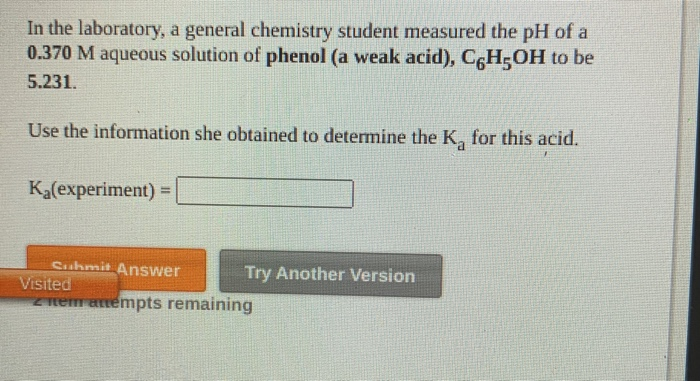In the laboratory, a general chemistry student measured the pH of a 0.370 M aqueous solution of phenol (a weak acid), C6H OH to be 5.231. Use the information she obtained to determine the K, for this acid. Ka(experiment) = Cushmit Answer Try Another Version Visited tremenempts remaining